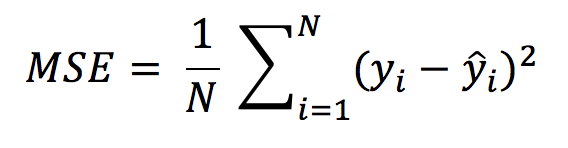# How do you find RMSE and MSE in a linear regression model?

How do you find RMSE and MSE in a linear regression model?

Mean Square Error is an absolute measure of the goodness for the fit.Mean Square Error formula

MSE is calculated by the sum of square of prediction error which is real output minus predicted output and then divide by the number of data points. It gives you an absolute number on how much your predicted results deviate from the actual number. You cannot interpret much insights from one single result but it gives you an real number to compare against other model results and help you select the best regression model.

Root Mean Square Error(RMSE) is the square root of MSE. It is used more commonly than MSE because firstly sometimes MSE value can be too big to compare easily. Secondly, MSE is calculated by the square of error, and thus square root brings it back to the same level of prediction error and make it easier for interpretation.

for more details on error metrics: ref:https://towardsdatascience.com/what-are-the-best-metrics-to-evaluate-your-regression-model-418ca481755b